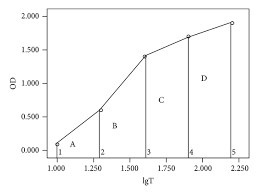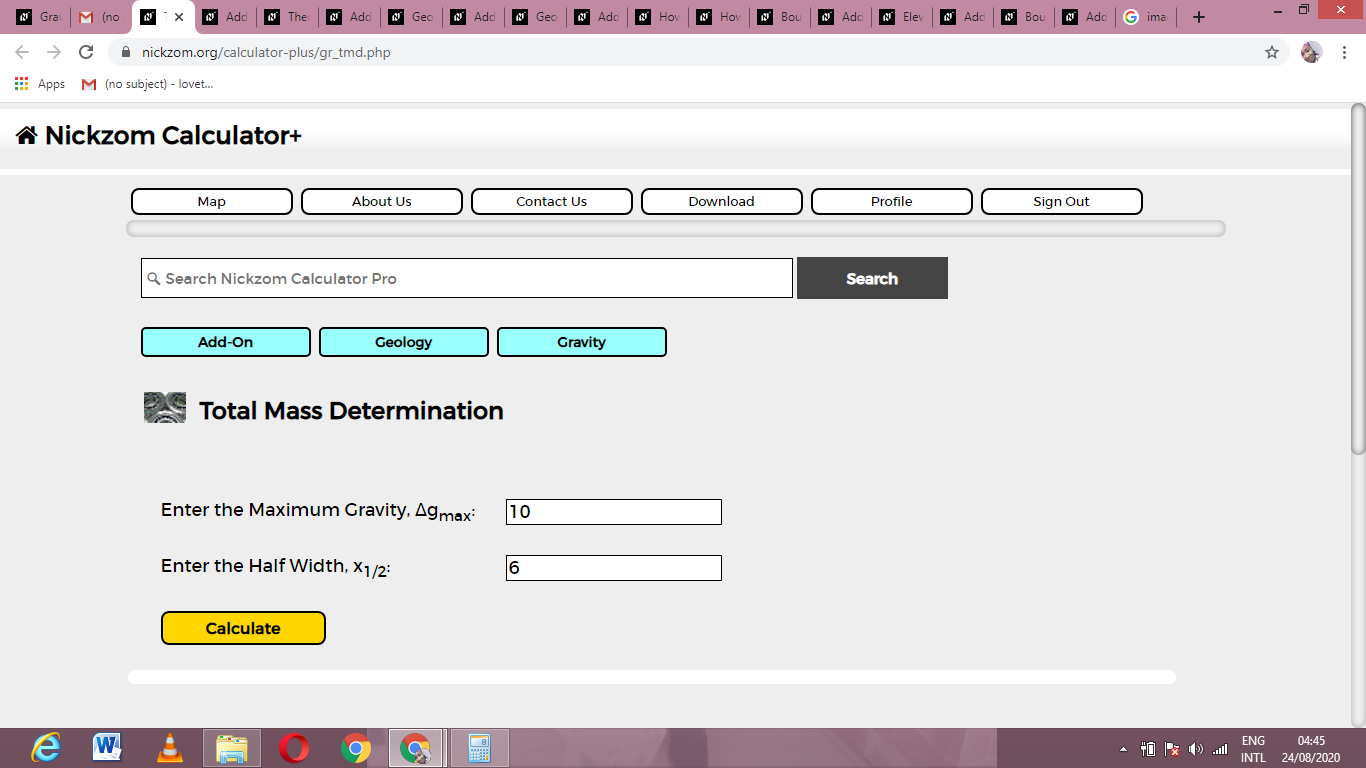# How to Calculate and Solve for Total Mass Determination | GravityThe image above represents total mass determination.

To compute for total mass determination, two essential parameters are needed and these parameters are Maximum Gravity (Δgmaxand Half Width (x1/2).

The formula for calculating total mass determination:

M ≃ 255Δgmax(x1/2

Where:

M = Total Mass
Δgmax = Maximum Gravity
x1/2 = Half Width

Let’s solve an example;
Find the total mass determination when the maximum gravity is 10 and the half width is 6.

This implies that;

Δgmax = Maximum Gravity = 10
x1/2 = Half Width = 6

M ≃ 255Δgmax(x1/2
M ≃ 255(10)(6)²
M ≃ 255(10)(36)
M ≃ 91800

Therefore, the total mass determination is 91800 kg.

Nickzom Calculator – The Calculator Encyclopedia is capable of calculating the total mass determination.

To get the answer and workings of the total mass determination using the Nickzom Calculator – The Calculator Encyclopedia. First, you need to obtain the app.

You can get this app via any of these means:

To get access to the professional version via web, you need to register and subscribe for NGN 2,000 per annum to have utter access to all functionalities.
You can also try the demo version via https://www.nickzom.org/calculator

Apple (Paid) – https://itunes.apple.com/us/app/nickzom-calculator/id1331162702?mt=8
Once, you have obtained the calculator encyclopedia app, proceed to the Calculator Map, then click on Geology under Add-on.Now, Click on Gravity under GeologyNow, Click on Total Mass Determination under GravityThe screenshot below displays the page or activity to enter your values, to get the answer for the total mass determination according to the respective parameters which are the Maximum Gravity (Δgmaxand Half Width (x1/2).Now, enter the values appropriately and accordingly for the parameters as required by the Maximum Gravity (Δgmaxis 10 and Half Width (x1/2) is 6.Finally, Click on CalculateAs you can see from the screenshot above, Nickzom Calculator– The Calculator Encyclopedia solves for the total mass determination and presents the formula, workings and steps too.